### Home > MC2 > Chapter 9 > Lesson 9.3.1 > Problem9-74

9-74.
1. The two triangles below are similar. Homework Help ✎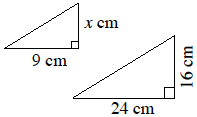1. Find x. Show your strategy.

2. What is the scale factor (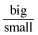)?

3. Find the area of each triangle.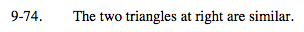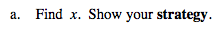Try finding the scale factor between the two corresponding sides that are given and solve for x.

Another way to solve for x is to create a proportion.
One possible proportion is shown below.

$\frac{24}{9} = \frac{16}{x}$

x = 6 cm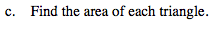Use the formula for area of a triangle:

$\frac{1}{2}(\text{base})(\text{height})$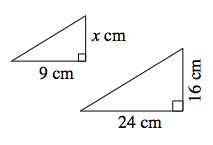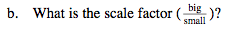What is the scale factor between the larger triangle and the smaller triangle? Find the ratio between corresponding sides.

Scale factor matches proportion.

$\frac{8}{3}$